# 专栏文章 弹窗识别_模型训练

~~~广告：打算组织个测试群分享活动，每周群内每个人轮流分享一次，大家互相监督 / 学习，共同提高，感兴趣的可以加进来：https://testerhome.com/topics/27429

## 背景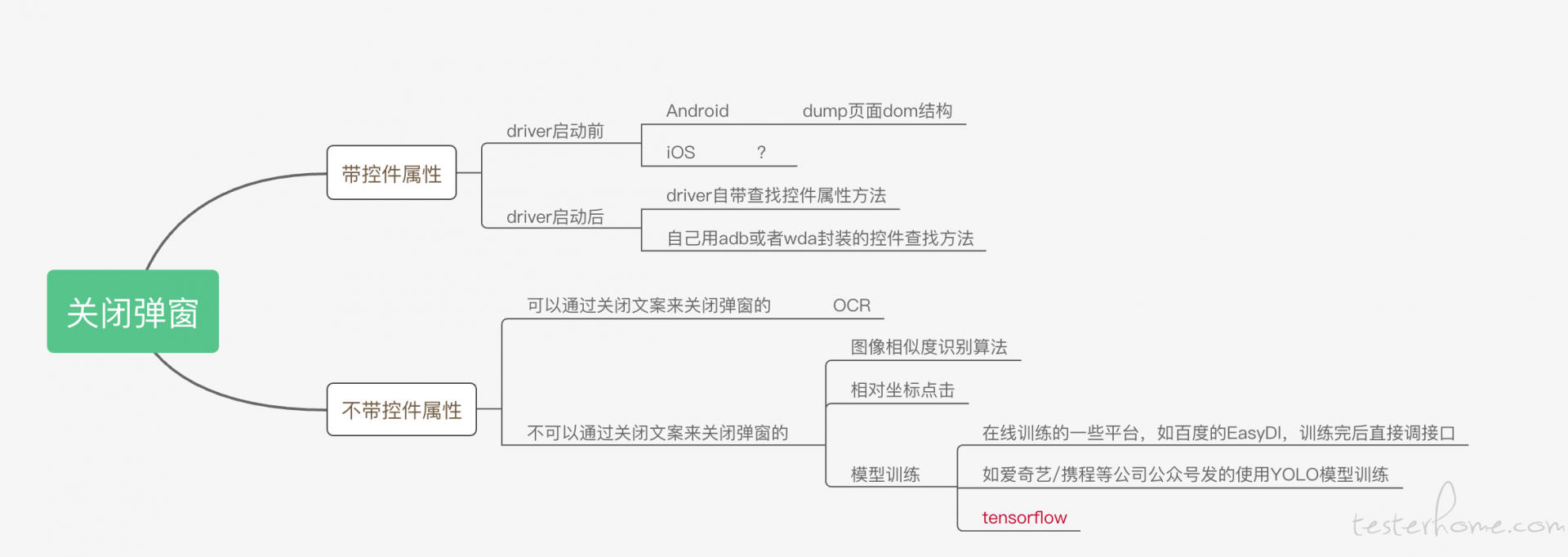## 名词解释

TensorFlow：是一个开源软件库，用于各种感知和语言理解任务的机器学习。
Keras：Keras 是一个用 Python 编写的开源神经网络库，能够在 TensorFlow、Microsoft Cognitive Toolkit、Theano 或 PlaidML 之上运行,进行深度学习模型的设计、调试、评估、应用和可视化。
CNN:卷积神经网络 (Convolutional Neural Networks)，是一类包含卷积计算且具有深度结构的前馈神经网络（Feedforward Neural Networks），是深度学习（deep learning）的代表算法之一。CNN 实际应用：图片分类、检索；目标定位检测；目标分割；人脸识别；骨骼识别。
R-CNN：属于 CNN 的一种，适用于图像检测、目标检测。

## 环境安装

``````Python3.7
Keras==2.2.4
numpy==1.16.5
tensorflow==1.15.2
scikit-learn==0.19.2
scipy==1.1.0
selectivesearch==0.4
opencv-python==3.4.2.17
h5py==2.10.0
``````

## 具体步骤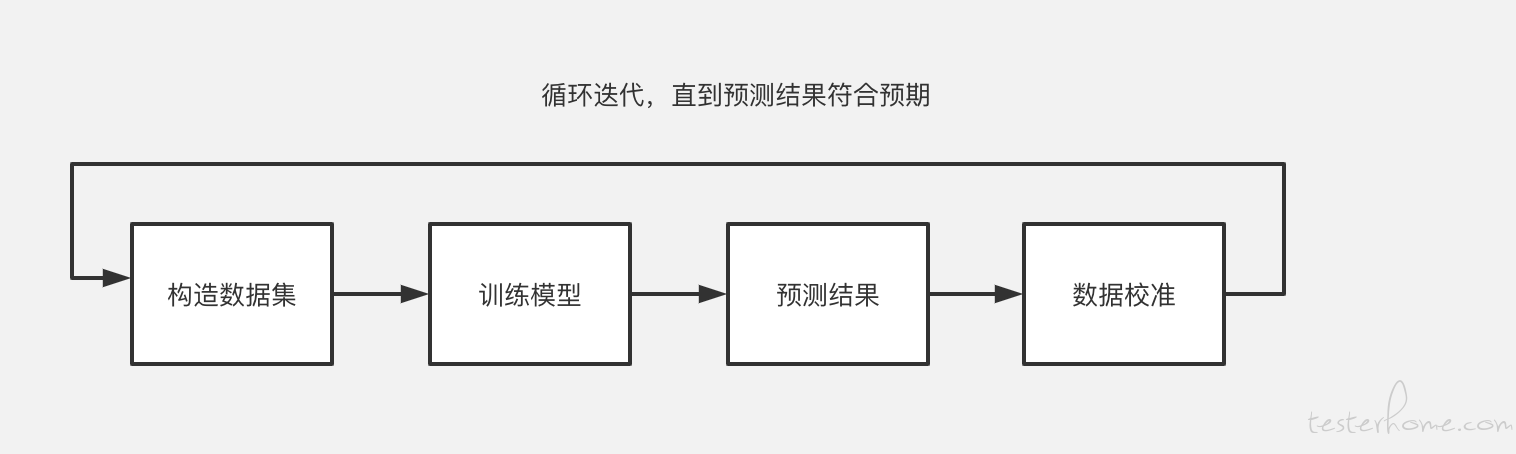1、构造数据集

• 正样本：11 个不同类型的活动弹窗关闭按钮图片• 负样本：11 个非活动弹窗关闭按钮图片``````data_gen = ImageDataGenerator(rotation_range=20, width_shift_range=0.1, height_shift_range=0.1, horizontal_flip=True,                              vertical_flip=True, fill_mode='nearest', data_format='channels_last')
``````

ImageDataGenerator() 通过实时数据增强生成张量图像数据批次，并且可以循环迭代，它是 keras.preprocessing.image 模块中的图片生成器，可以每一次给模型 “喂” 一个 batch_size 大小的样本数据，同时也可以在每一个批次中对这 batch_size 个样本数据进行增强，扩充数据集大小，增强模型的泛化能力。比如进行旋转，变形，归一化等等。

（1）图片生成器，负责生成一个批次一个批次的图片，以生成器的形式给模型训练；
（2）对每一个批次的训练图片，适时地进行数据增强处理（data augmentation）；

``````for _ in data_gen.flow(x, batch_size=1, save_to_dir=self.train_path, save_prefix=cls_prefix, save_format="png"):
i = i+1
if i >= augmentation_size:
print("class_{0} augmentation for {1} samples".format(cls_prefix, i))
break
flow(self, X, y, batch_size=32, shuffle=True, seed=None, save_to_dir=None, save_prefix='', save_format='png')：
``````

2、训练模型

Sequential 序贯模型
Sequential 序贯模型是函数式模型的简略版，为最简单的线性、从头到尾的结构顺序，不分叉，是多个网络层的线性堆叠。Keras 实现了很多层，包括 core 核心层，Convolution 卷积层、Pooling 池化层等非常丰富有趣的网络结构。我们可以通过将层的列表传递给 Sequential 的构造函数，来创建一个 Sequential 模型。

``````model = Sequential()
``````

``````model.add(MaxPooling2D(pool_size=(2, 2)))
``````

• 损失函数 loss：模型试图最小化的目标函数。它可以是现有损失函数的字符串标识符，如 categorical_crossentropy 或 mse，也可以是一个目标函数。详见：losses。
• 评估标准 metrics：对于任何分类问题，你都希望将其设置为 metrics = ['accuracy']。评估标准可以是现有的标准的字符串标识符，也可以是自定义的评估标准函数。

``````model.compile(loss=keras.losses.categorical_crossentropy,
metrics=['accuracy'])
``````

Keras 模型在输入数据和标签的 Numpy 矩阵上进行训练。为了训练一个模型，你通常会使用 fit 函数

``````history = model.fit(x_train, y_train,
batch_size=batch_size,
epochs=epochs,
verbose=1,
validation_data=(x_test, y_test))
``````

3、预测结果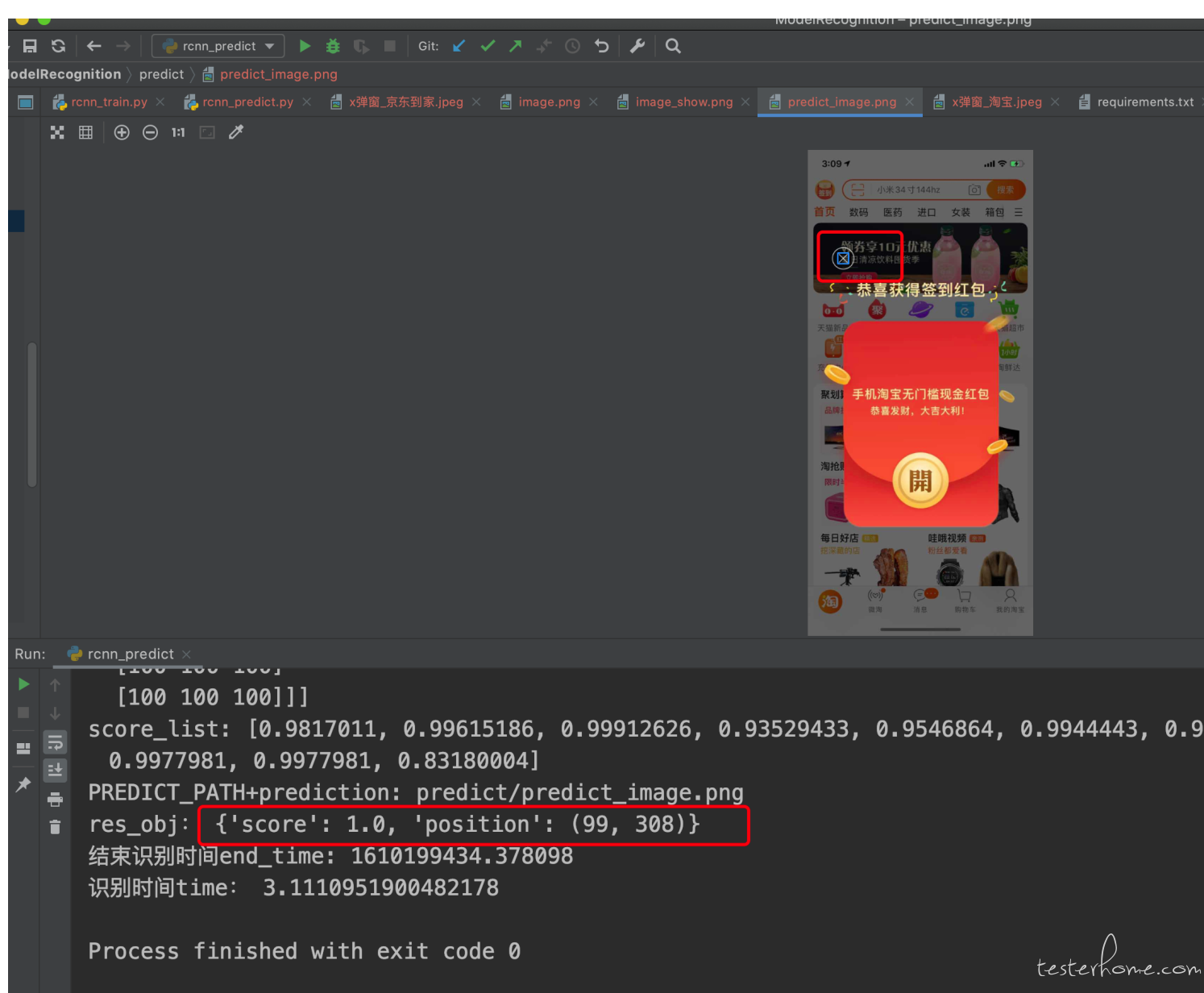4、模型评价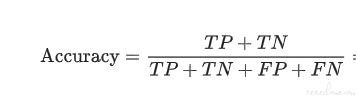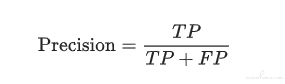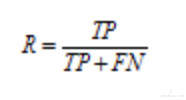## 参考文档